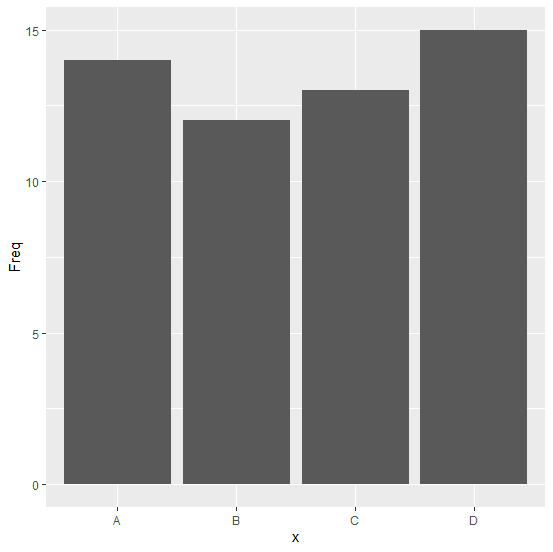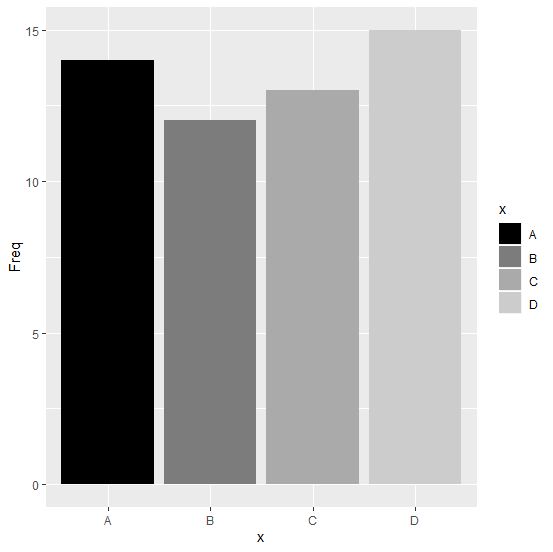# How to change the bars color to grey shade of a bar graph created by using ggplot2 in R?

When we create a bar graph using ggplot2, the color of the bars is dark grey but it can be changed to different colors or we can also give different shades of grey to them. This will be helpful if we are plotting a pattern of categorical data. For example, plotting educational level on X-axis with frequencies of years of experience on Y-axis. We can do this by using scale_fill_grey function of ggplot2 package.

## Example

Consider the below data frame −

Live Demo

> x<-c("A","B","C","D")
> Freq<-c(14,12,13,15)
> df<-data.frame(x,Freq)
> df

## Output

 x Freq
1 A 14
2 B 12
3 C 13
4 D 15
> library(ggplot2)
> ggplot(df,aes(x,Freq))+geom_bar(stat="identity")Giving grey shades to bars −

>
ggplot(df,aes(x,Freq))+geom_bar(stat="identity",aes(fill=x))+scale_fill_grey(start=0,end =0.8)

## Output